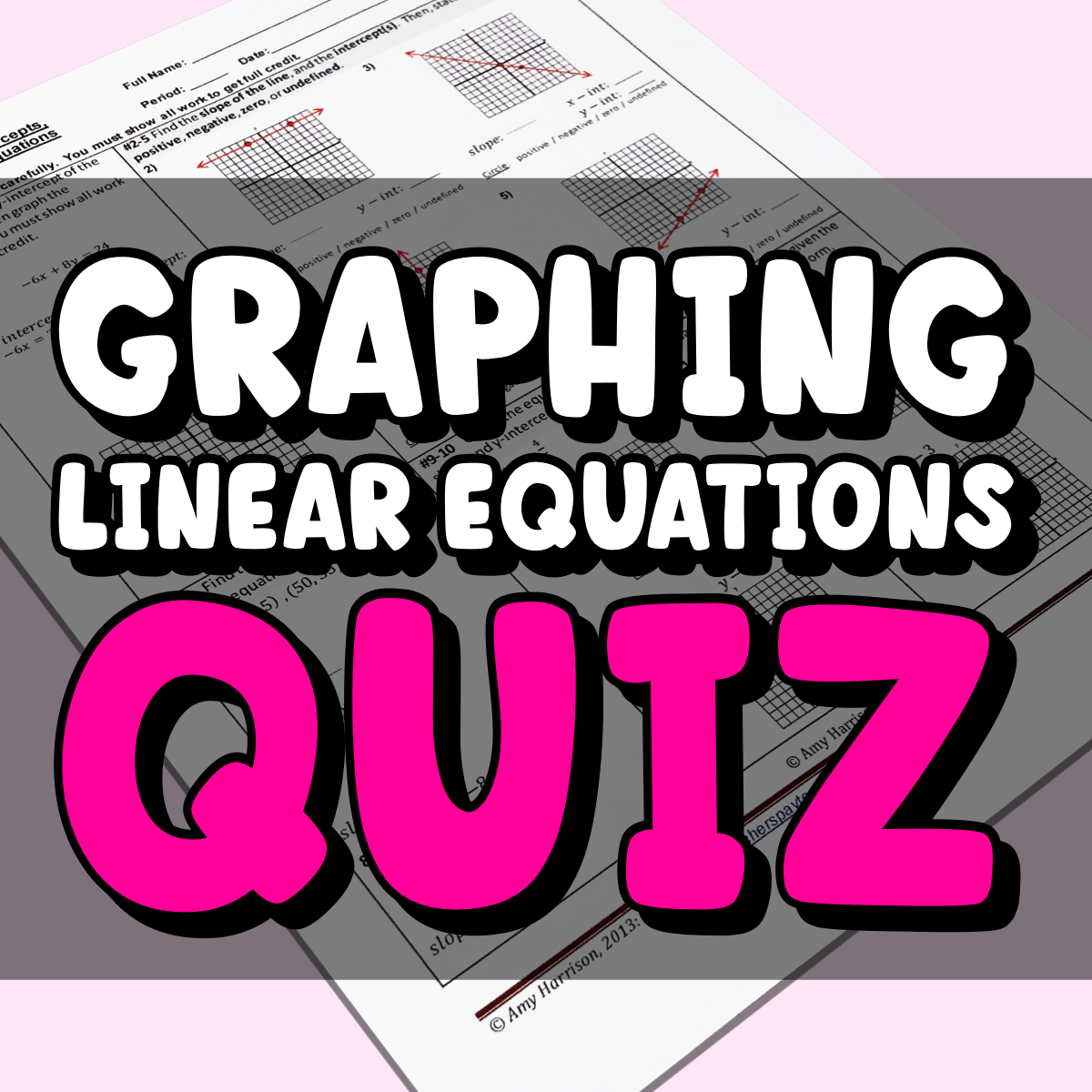# Slope, Graphing Linear Equations, and x & Y Intercepts Quiz

## Do you need a slope, graphing linear equations, & x and y intercepts quiz for your middle school math class?

Don’t spend your precious free time creating your own linear equations quiz.

We did the hard work for you!

• 12 Questions

## Topics Covered in Quiz:

• Find the x- and y- intercepts given an equation.
• Find the slope of the line, and find the intercepts.
• Find the slope of the line given two points
• Graph the equation given the slope and y-intercept
• Graph the equation given a linear equation in slope-intercept form.

The graphs print and copy well. The lines are dark and clear. The thumbnails do not do them justice.

## Other teachers LOVE this resource.

Check out two of the dozens of perfect reviews:

Great variety of quiz topics- covers all aspects of linear equations- great product!

-Judy H.

I love that I didn’t have to create my own document with graphs in order for my students to practice finding and creating slope. Can’t wait to use this!

-Valerie W.